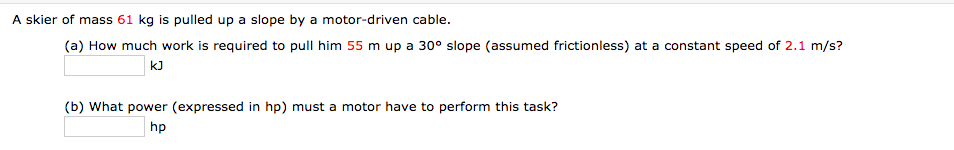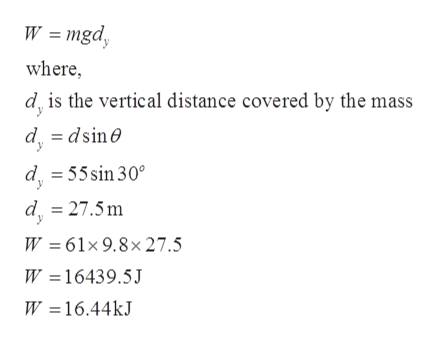# A skier of mass 61 kg is pulled up a slope by a motor-driven cable.(a) How much work is required to pull him 55 m up a 30° slope (assumed frictionless) at a constant speed of 2.1 m/s?kJ(b) What power (expressed in hp) must a motor have to perform this task?hp

Question
7 viewshelp_outlineImage TranscriptioncloseA skier of mass 61 kg is pulled up a slope by a motor-driven cable. (a) How much work is required to pull him 55 m up a 30° slope (assumed frictionless) at a constant speed of 2.1 m/s? kJ (b) What power (expressed in hp) must a motor have to perform this task? hp fullscreen
check_circle

Step 1

Given:

Mass = 61 kg

Distance to be covered = 55 m

Angle = 30o

Speed = 2.1 m/s

Step 2

Part (a): Work done can be calculated as:help_outlineImage TranscriptioncloseW = mgd, where, d is the vertical distance covered by the mass d = dsine d = 55 sin 30° d = 27.5 m W = 61×9.8× 27.5 W =16439.5J W =16.44kJ fullscreen
Step 3

Time taken by the skier to trav...

### Want to see the full answer?

See Solution

#### Want to see this answer and more?

Solutions are written by subject experts who are available 24/7. Questions are typically answered within 1 hour.*

See Solution
*Response times may vary by subject and question.
Tagged in

### Work,Power and Energy